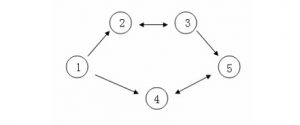<h1>题目</h1>

<h2>题目描述</h2>

$C$ 国有 $n$ 个大城市和 $m$ 条道路，每条道路连接这 $n$ 个城市中的某两个城市。任意两个城市之间最多只有一条道路直接相连。这 $m$ 条道路中有一部分为单向通行的道路，一部分为双向通行的道路，双向通行的道路在统计条数时也计为 1 条。

$C$ 国幅员辽阔，各地的资源分布情况各不相同，这就导致了同一种商品在不同城市的价格不一定相同。但是，同一种商品在同一个城市的买入价和卖出价始终是相同的。<h2>输入格式</h2>

<h2>输出格式</h2>

<h2>样例输入</h2>

5 5
4 3 5 6 1
1 2 1
1 4 1
2 3 2
3 5 1
4 5 2


<h2>样例输出</h2>

5


<h1>解题报告</h1>

<h1>样例代码</h1>

#include <iostream>
#include <cstdio>
#include <stack>
#include <cstring>
#include <queue>

const int MAXN = 100000 + 5;
const int MAXM = 500000 + 5;

struct Node;
struct Edge;

int N,M;

Edge New_Graph(Node ,Node *);
Edge New_Scc(Node ,Node *);

int in[MAXN],tot,dist[MAXN],dfn[MAXN],low[MAXN],cor[MAXN];
int mincost[MAXN],maxcost[MAXN],ans[MAXN];

struct Node{
int num;
bool instack;
Edge *firstEdge;
}node[MAXN],scc[MAXN];

struct Edge{
Node s,t;
Edge *next;
}Graph_pool[MAXM  2],Graph_frog = Graph_pool,Scc_pool[MAXM  2],Scc_frog = Scc_pool;

Edge New_Graph(Node u,Node *v){
Edge *ret = ++Graph_frog;
ret->s = u;ret->t = v;
ret->next = u->firstEdge;
return ret;
}

Edge New_Scc(Node u,Node *v){
Edge *ret = ++Scc_frog;
ret->s = u;ret->t = v;
ret->next = u->firstEdge;
return ret;
}

node[u].firstEdge = New_Graph(&node[u],&node[v]);
}

scc[u].firstEdge = New_Scc(&scc[u],&scc[v]);
}

void init(){
for(int i = 1;i <= N;i++){
scc[i].num = node[i].num = i;
node[i].instack = false;
}
memset(mincost,0x3f,sizeof(mincost));
}

std::stack<Node *> s;
int timer = 0;

inline void tarjan(Node *v){
dfn[v->num] = low[v->num] = ++timer;
s.push(v);
v->instack = true;
for(Edge *e = v->firstEdge;e;e = e->next){
if(!dfn[e->t->num]){
tarjan(e->t);
low[v->num] = std::min(low[v->num],low[e->t->num]);
}
else
if(e->t->instack)
low[v->num] = std::min(low[v->num],dfn[e->t->num]);
}
if(dfn[v->num] == low[v->num]){
Node *r; ++tot;
do{
r = s.top();s.pop();
r->instack = false;
cor[r->num] = tot;
maxcost[tot] = std::max(maxcost[tot],dist[r->num]);
mincost[tot] = std::min(mincost[tot],dist[r->num]);
}while(r != v);
}
}

void Rebuild(){
for(int i = 1;i <= N;i++){
for(Edge *e = node[i].firstEdge;e;e = e->next){
if(cor[i] == cor[e->t->num] || cor[e->t->num] == cor || cor[N] == cor[i])
continue;
++in[cor[i]];
}
}
}

void topsort(){
std::queue<Node *> q;
for(int i = 1;i <= tot;i++)
if(!in[i] && i != cor[N])
q.push(&scc[i]);
while(!q.empty()){
Node *v = q.front();q.pop();
for(Edge *e = v->firstEdge;e;e = e->next){
if(!--in[e->t->num])
q.push(e->t);
}
}
q.push(&scc[cor[N]]);
while(!q.empty()){
Node *v = q.front();q.pop();
ans[v->num] = std::max(ans[v->num],maxcost[v->num] - mincost[v->num]);
for(Edge *e = v->firstEdge;e;e = e->next){
if(!--in[e->t->num])
q.push(e->t);
maxcost[e->t->num] = std::max(maxcost[v->num],maxcost[e->t->num]);
ans[e->t->num] = std::max(ans[e->t->num],ans[v->num]);
}
}
}

int main(){
scanf("%d%d",&N,&M);
init();
for(int i = 1;i <= N;i++)
scanf("%d",&dist[i]);
for(int u,v,opt,i = 1;i <= M;i++){
scanf("%d%d%d",&u,&v,&opt);
if(opt == 2)